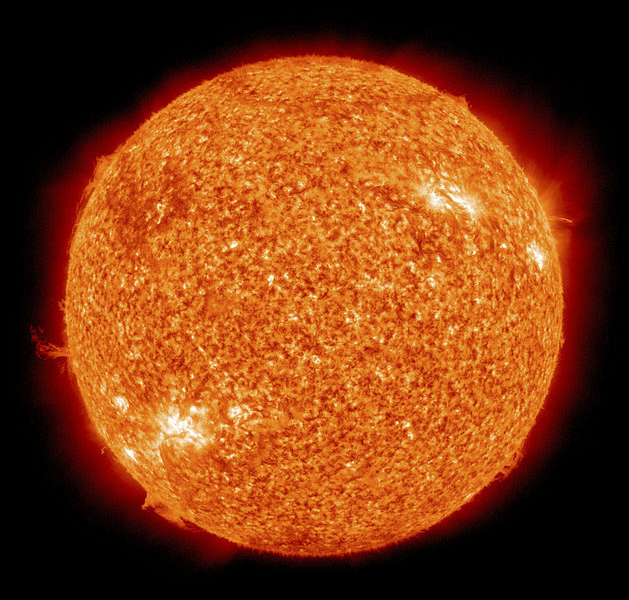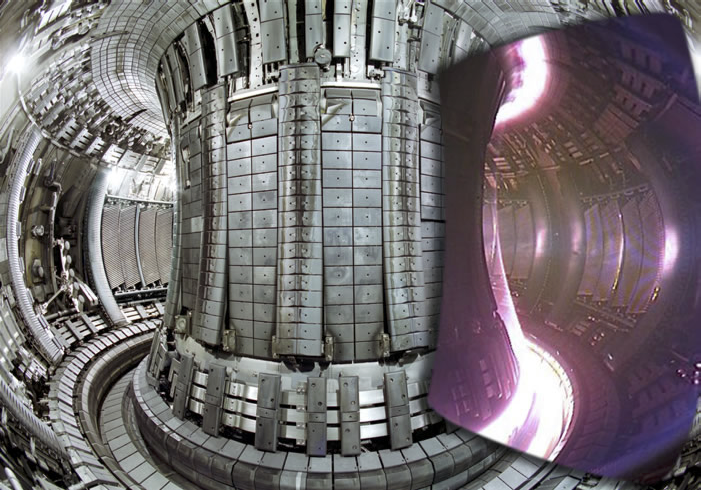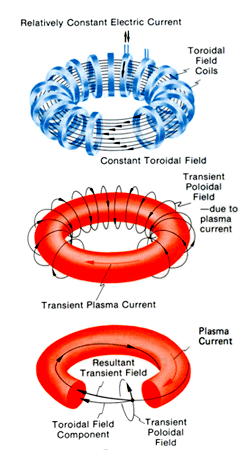## Section57.6Nuclear Fusion

Nuclear fusion occurs when two or more smaller nuclei fuse together to form a larger nucleus which has less mass than the combined masses of the reactants. In Figure 57.1.3 of binding energy per nucleon plot we notice that the binding energy per nucleon increases with increasing mass number $A$ up to $A=60\text{.}$ Therefore, when smaller nuclei combine to make a larger nucleus, the difference in the binding energy will be released in the reaction. The energy in hydrogen-rich stars such as the Sun is driven by the fusion process (Figure 57.6.1).Figure 57.6.1. The sun is a fusion reactor deriving energy from nuclear reactions.

For instance, it is believed that the following chain of fusion reactions, called the proton-proton chain - I, occurs in the Sun.

\begin{align*} \amp \textrm{p} + \textrm{p} \longrightarrow\ ^{2}H + e^{+} + \nu_e\\ \amp ^{2}\textrm{H} + \textrm{p} \longrightarrow\ ^{3}\textrm{He} + \gamma \\ \amp ^{3}\textrm{He} + ^{3}\textrm{He} \longrightarrow\ ^{4}\textrm{He} + \textrm{p} + \textrm{p} \end{align*}

In the first reaction, two protons are fused to make a deuteron, a positron, and a neutrino. In the second reaction, the deuteron fuses with another proton with the production of $^{3}\textrm{He}\text{.}$ Two $^{3}\textrm{He}$ then combine to form $^{4}\textrm{He}$ and release two protons. Thus, two times the first two reactions and one time the third equation forms a full cycle. In the full chain, four protons combine in the first reaction and two in the second reaction, and two protons are released in the third reaction. Thus, a total of four protons combine to yield one $^{4}\textrm{He}\text{,}$ two positrons, two neutrino, and two photons.

The net energy released as positron, neutrino, gamma rays, and kinetic energy of $^4$He over one proton-proton chain will be

\begin{equation*} Q_{\textrm{net}} = \left(4 m_p - m[\ ^{4}\textrm{He}] \right)\: c^2\approx 25\:\textrm{MeV}. \end{equation*}

When the two positrons released in the p-p chain collide with two electrons in the Sun, they annihilate each other and produce gamma rays with additional energy of approximately 2 MeV. This makes the total energy released from the proton-proton chain plus annihialtion of positron to be about 27 MeV per four proton. The proton-proton chain reaction occurs deep inside the Sun where the temperature is quite high, $\sim 15\text{ MK}\text{.}$

It is difficult to bring two protons close enough to fuse due to enormous Coulomb repulsion between them. However, because of the potential payoff of energy from fusion reactions, there is continued search for creating a controlled and sustained nuclear fusion. To overcome the Coulomb repulsion and bring two protons to within a distance of 1 fm we will need to give the protons a kinetice energy equal to the potential energy when they are 1 fm apart, which will be

\begin{equation*} U [1\:\textrm{fm}] = k \frac{e^2}{r} = 9\times 10^9 \frac{\left(1.6\times 10^{-19}\right)^2}{10^{-15}} = 1.44\:\textrm{MeV}. \end{equation*}

This energy will come from the kinetic energies of the protons. Suppose protons are produced by heating hydrogen to a temperature $T\text{,}$ then each proton must have half this potential energy so that the total kinetic energy when they are far apart will equal to the total potential energy when they are closest.

\begin{equation*} K_{\textrm{each}} = 0.72\:\textrm{MeV} \end{equation*}

Equating this to the thermal energy for each particle gives us a clue about the temperature we need.

\begin{equation*} \frac{3}{2}k_B T = 0.72\:\textrm{MeV}. \end{equation*}

With $k_B = 8.61733 \times 10−{11} \text{ MeV/K}\text{,}$ we get

\begin{equation*} T = 5.57 \times 10^9\:\textrm{K}. \end{equation*}

### Subsection57.6.1Controlled Fusion

Controlled fission has been used to build nuclear power plants based on the fission of $^{235}$U. The by products of fission are long-lived radioactive material whose disposal is a major problem. Fusion of small nuclei such as deuterium or tritium will produce short-lived isotopes $^3$H or stable nuclei $^4$He and would be preferable over fission plants. The most common reaction tried in modern fusion reactors use the following fusion reaction between deterium and tritium, called the D-T fusion.

\begin{equation*} ^{2}\textrm{H} + ^{3}\textrm{H} \longrightarrow\ ^{4}\textrm{He} + \textrm{n}\ \ \ (Q = 17.6\:\textrm{MeV}) \end{equation*}

Of the 17.6 MeV energy released, the neutron carries off 80\% of the energy and alpha particle the remaining 20\%. For a fusion nuclear reactor to be successful in its operations, we need to have high density of the reactants $\rho\text{,}$ high temperature $T$ to provide enough kinetic energy, and a long time for the nuclei to be confined within a small space, called the confinement time, $\tau\text{.}$ The requirements of a fusion reactor are expressed in terms of the product of $\rho\text{,}$ $T\text{,}$ and $\tau\text{,}$ called the Lawson's criterion.

\begin{equation*} \rho k_BT \tau \geq 5\times 10^{18}\: \textrm{MeV.s/m}^2. \end{equation*}

Two mechanisms of confinement have been attempted - magnetic confinement and inertial confinement. In a magnetic confinement, magnetic field is used to control the particles and in the inertial confinement particles are struck with laser beams to heat them as well as bring them closer.

#### Subsubsection57.6.1.1Magnetic Confinement

The magnetic confinement works on the principle that moving charged particles experience magnetic force. A torroidal geometry magnetic confinement system called tokamak, developed in Russia and implemented at several sited around the world has seen some success in meeting the Lawson's criterion. Russians first reported quasistationary thermonuclear fusion reaction in 1968, but in the west, it is believed that the first truly controlled fusion power was achieved in 1991 at the Joint European Torus in Oxfordshire, England.

In a tokamak, a torroidal space is evacuated and two sources of magnetic field act on the particles moving near the center of the chamber (see Figure 57.6.2. A stationary magnetic field, called the torroidal field $B_{\phi}$ is produced by a steady current in wires wound around the torus. This field goes around the circle in the torroidal space. A second magnetic field, called transient poloidal field $B_p\text{,}$ is induced by the transient plasma current. The net field is a helical field around the plasma current as illustrated in Figure 57.6.3.Figure 57.6.2. Internal view of the JET tokamak. This image maybe copyrighted by JET. Credits: Wikicommon media.Figure 57.6.3. The torroidal and poloidal magnetic fields, and the resulting helical magnetic field in a Tokamak. Credits: Wikicommon media.

An international nuclear research facility called International Thermonuclear Experimental Reactor (ITER) is under construction in 2014, at Cadarache facility at a cost of 12 billion dollars in south France. ITER is expected to produce 500 MW power from fusion. ITER will also work with the D-T fusion. The full operation of the facility is expected to start in 2027. Other notable research facilities for fusion has been the Tokamak Fusion Test Reactor (TFTR) which was an experimental tokamak built at Princeton Plasma Physics Laboratory and operated from 1982 to 1997.

ITER is expected to operate at the temperature such that $k_BT = 10\:\textrm{keV}\text{.}$ (a) Find the temperature. (b) Find the density needed if a confinement time of 10 ms is desired.

Hint

Use $k_BT\text{.}$

(a) $1.2\times 10^{8}\:\textrm{K}\text{,}$ (b) $5\times 10^{22}\: \textrm{per m}^3\text{.}$

Solution

(a) From the given data

\begin{equation*} T = \frac{10\:\textrm{keV}}{k_B} = \frac{10\:\textrm{keV}}{8.62\times10^{-8}\textrm{keV/K}} = 1.2\times 10^{8}\:\textrm{K}. \end{equation*}

(b) From Lawson's criterion we get

\begin{equation*} \rho \geq \frac{5\times 10^{18}\: \textrm{MeV.s/m}^3}{\tau k_BT}. \end{equation*}

Using $k_B T = 0.01$ MeV and $\tau = 0.01$ s in the denominator we get

\begin{equation*} \rho \geq \frac{5\times 10^{18}\: \textrm{MeV.s/m}^3}{0.01\:\textrm{s} \times 0.01\:\textrm{MeV}} = 5\times 10^{22}\: \textrm{per m}^3. \end{equation*}

#### Subsubsection57.6.1.2Inertial Confinement

An alternative to the magnetic confinement is an inertial confinement in which a fuel target, usually a pellet, is heated and compressed usually by lasers. The pellet is usually made of deuterium or deuterium-tritium mixture. The heating of the outer layer causes it to explode sending a compressing wave to the center of the pellet where the high pressure and temperature builds up which is expected to cause fusion reaction.

A major undertaking is the National Ignition Facility (NIF) at Lawrence Livermore National Laboratory (LLNL) in USA. Two earlier versions of the project, named Shiva and NOVA, were unsuccessful in ignition, but much physics about the nuclear explosion process was learned which is being used in the new NIF faciltiy. In the NIF facility, 192 laser beams are directed inside a gold cylinder, called a hohlraum, which contains a small fuel pellet of deuterium and tritium. Each laser beam generates enormous power of about 500 trillion Watts in small pulses of width 20 billionths of a second. The energy of the lasers are deposited at the surface of the pellet. For a brief moment the nuclei in the pellet are expected to fuse and produce a “miniature star” and produce the fusion energy.

TODO: \​begin{exercise} For two deuterons to fuse into a triton and a proton the two deuterons must come within a distance of 10 fm. (a) What is the electrical repulsive force between the two deuterons at that distance? (b) What should be the kinetic energies of the two deuterons if they are to overcome the repulsive force? (c) What should be temperature of the plasma if the deuterons are to have the required kinetic energy? \end{exercise}